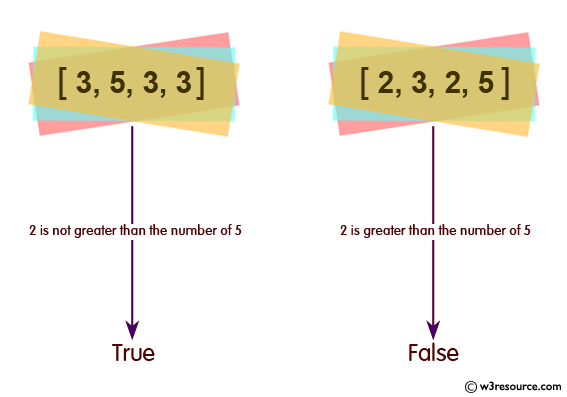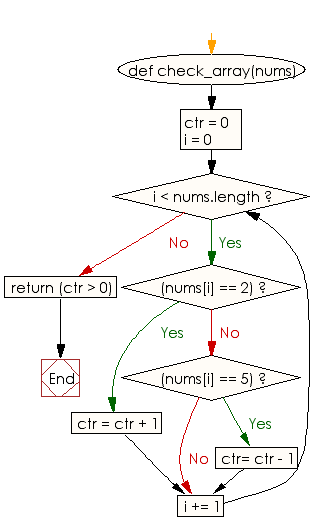﻿ Ruby Array exercises: Check whether the number of 2's is greater than the number of 5's of an given array of integers - w3resource# Ruby Array Exercises: Check whether the number of 2's is greater than the number of 5's of a given array of integers

## Ruby Array: Exercise-34 with Solution

Write a Ruby program to check whether the number of 2's is greater than the number of 5's of a given array of integers.Ruby Code:

``````def check_array(nums)
ctr = 0
i = 0
while i < nums.length
if (nums[i] == 2)
ctr = ctr + 1
elsif (nums[i] == 5)
ctr= ctr - 1
end
i += 1
end
return (ctr > 0)
end
print check_array([3, 5, 3, 3]),"\n"
print check_array([2, 3, 2, 5]),"\n"
print check_array([2, 2, 5, 5]),"\n"
``````

Output:

```false
true
false
```

Flowchart:Ruby Code Editor: